## 理论

### 霍夫线变换：

1. 霍夫线变化是用于检测直线的一种变化。
2. 为了运用这种变化，您需要首先进行一个边缘检测预处理

#### 它是如何工作的？

1. 众所周知, 一条直线在图像二维空间可由两个变量表示. 例如:

1.在 笛卡尔坐标系: 可由参数:斜率和截距表示.

2.在 极坐标系: 可由参数:极径和极角表示赵君沛然 3年前

1. 一般来说对于点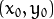, 我们可以将通过这个点的一族直线统一定义为: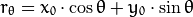这就意味着每一对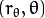代表一条通过点的直线.

2. 如果对于一个给定点我们绘出所有通过它的直线, 将得到一条正弦曲线. 例如, 对于给定点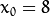and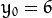我们可以绘出下图 (在平面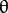-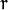):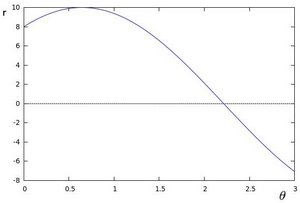只考虑满足下列条件的点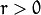and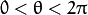.

3. 我们可以对图像中所有的点进行上述操作. 如果两个不同点进行上述操作后得到的曲线在平面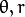相交, 这就意味着它们通过同一条直线. 例如, 接上面的例子我们继续对点: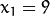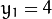和点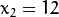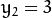绘图, 得到下图: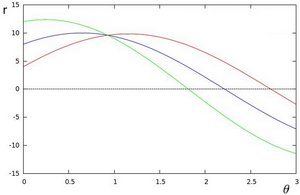这三条曲线在平面相交于点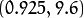, 坐标表示的是参数对 (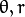) 或者是说点, 点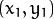和点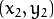组成的平面内的的直线.

4. 那么以上的内容要说明什么呢? 这意味着一般来说, 这意味着一般来说，可以通过找到曲线之间的交叉点的数量来检测 线.。越多曲线交于一点也就意味着这个交点表示的直线由更多的点组成. 一般来说我们可以通过设置直线上点的 阈值 来定义多少条曲线交于一点我们才认为 检测 到了一条直线.

5. 这就是霍夫线变换要做的. 它追踪图像中每个点对应曲线间的交点. 如果交于一点的曲线的数量超过了 阈值, 那么可以认为这个交点所代表的参数对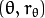在原图像中为一条直线.toypipi 2年前

#### 标准霍夫线变换和统计概率霍夫线变换

OpenCV实现了以下两种霍夫线变换:

1. 标准霍夫线变换
• 原理在上面的部分已经说明了. 它能给我们提供一组参数对的集合来表示检测到的直线
• 在OpenCV 中通过函数 HoughLines 来实现
1. 统计概率霍夫线变换
• 这是执行起来效率更高的霍夫线变换. 它输出检测到的直线的端点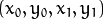• 在OpenCV 中它通过函数 HoughLinesP 来实现

## 代码

1. 这个程序是用来做什么的?
• 加载一幅图片
• 对图片进行 标准霍夫线变换 或是 统计概率霍夫线变换.
• 分别在两个窗口显示原图像和绘出检测到直线的图像.
2. 我们将要说明的例程能从 这里 下载。 一个更高级的版本 (能同时演示标准霍夫线变换和统计概率霍夫线变换并带有活动条来改变变换的阈值) 能从 这里 下载。卓小混混 3年前
#include "opencv2/highgui/highgui.hpp"
#include "opencv2/imgproc/imgproc.hpp"

#include <iostream>

using namespace cv;
using namespace std;

void help()
{
cout << "\nThis program demonstrates line finding with the Hough transform.\n"
"Usage:\n"
"./houghlines <image_name>, Default is pic1.jpg\n" << endl;
}

int main(int argc, char** argv)
{
const char* filename = argc >= 2 ? argv : "pic1.jpg";

Mat src = imread(filename, 0);
if(src.empty())
{
help();
cout << "can not open " << filename << endl;
return -1;
}

Mat dst, cdst;
Canny(src, dst, 50, 200, 3);
cvtColor(dst, cdst, CV_GRAY2BGR);

#if 0
vector<Vec2f> lines;
HoughLines(dst, lines, 1, CV_PI/180, 100, 0, 0 );

for( size_t i = 0; i < lines.size(); i++ )
{
float rho = lines[i], theta = lines[i];
Point pt1, pt2;
double a = cos(theta), b = sin(theta);
double x0 = a*rho, y0 = b*rho;
pt1.x = cvRound(x0 + 1000*(-b));
pt1.y = cvRound(y0 + 1000*(a));
pt2.x = cvRound(x0 - 1000*(-b));
pt2.y = cvRound(y0 - 1000*(a));
line( cdst, pt1, pt2, Scalar(0,0,255), 3, CV_AA);
}
#else
vector<Vec4i> lines;
HoughLinesP(dst, lines, 1, CV_PI/180, 50, 50, 10 );
for( size_t i = 0; i < lines.size(); i++ )
{
Vec4i l = lines[i];
line( cdst, Point(l, l), Point(l, l), Scalar(0,0,255), 3, CV_AA);
}
#endif
imshow("source", src);
imshow("detected lines", cdst);

waitKey();

return 0;
}CY2 3年前

## 说明

1. 加载图片

Mat src = imread(filename, 0);
if(src.empty())
{
help();
cout << "can not open " << filename << endl;
return -1;
}

2. 用Canny算子对图像进行边缘检测

Canny(src, dst, 50, 200, 3);


现在我们将要执行霍夫线变换. 我们将会说明怎样使用OpenCV的函数做到这一点:

3. 标准霍夫线变换

1. 首先, 你要执行变换:

vector<Vec2f> lines;
HoughLines(dst, lines, 1, CV_PI/180, 100, 0, 0 );


有以下自变量:

• dst: 边缘检测的输出图像. 它应该是个灰度图 (但事实上是个二值化图)
• lines: 储存着检测到的直线的参数对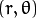的容器
• rho : 参数的分辨率（以像素为单位）。 我们使用1像素。
• theta: 参数以弧度为单位的分辨率. 我们使用 1度 (即CV_PI/180)
• threshold: 要”检测” 一条直线所需最少的的曲线交点
• srn and stn: 参数默认为0. 查阅OpenCV参考文献来获取更多信息.
2. 然后通过画出检测到的直线来显示结果.

for( size_t i = 0; i < lines.size(); i++ )
{
float rho = lines[i], theta = lines[i];
Point pt1, pt2;
double a = cos(theta), b = sin(theta);
double x0 = a*rho, y0 = b*rho;
pt1.x = cvRound(x0 + 1000*(-b));
pt1.y = cvRound(y0 + 1000*(a));
pt2.x = cvRound(x0 - 1000*(-b));
pt2.y = cvRound(y0 - 1000*(a));
line( cdst, pt1, pt2, Scalar(0,0,255), 3, CV_AA);
}

4. 统计概率霍夫线变换

1. 首先, 你要执行变换:

vector<Vec4i> lines;
HoughLinesP(dst, lines, 1, CV_PI/180, 50, 50, 10 );


有以下自变量:

• dst: 边缘检测的输出图像. 它应该是个灰度图 (但事实上是个二值化图)
• lines: 储存着检测到的直线的参数对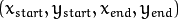的容器
• rho : 参数的分辨率（以像素为单位）。 我们使用1像素。
• theta: 参数以弧度为单位的分辨率. 我们使用 1度 (即CV_PI/180)
• threshold: 要”检测” 一条直线所需最少的的曲线交点
• minLinLength: 能组成一条直线的最少点的数量. 点数量不足的直线将被抛弃.
• maxLineGap: 能被认为在一条直线上的亮点的最大距离.
2. 通过画出检测到的直线来显示结果.

for( size_t i = 0; i < lines.size(); i++ )
{
Vec4i l = lines[i];
line( cdst, Point(l, l), Point(l, l), Scalar(0,0,255), 3, CV_AA);
}

5. 显示原始图像和检测到的直线:

imshow("source", src);
imshow("detected lines", cdst);

6. 等待用户按键推出程序

waitKey();toypipi 2年前

## 结果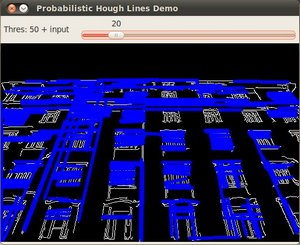卓小混混 3年前